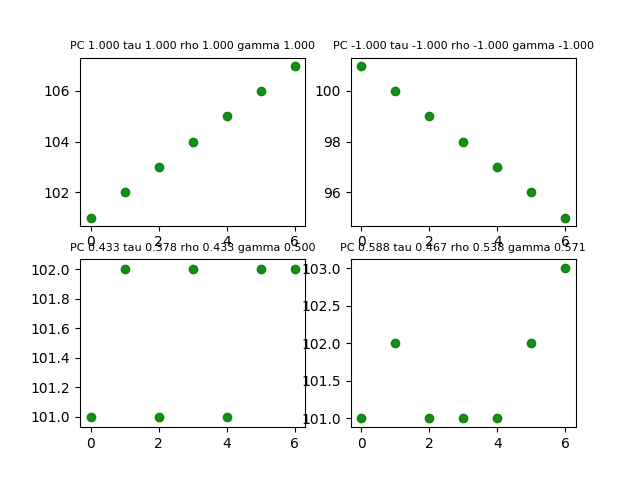# What is Correlation ?

Correlation is  used to indicate dependence or association is any statistical relationship, whether causal or not, between two random variables or bivariate data. It is a measure of relationship between two mathematical variables or measured data values, which includes the Pearson correlation coefficient as a special case.Correlation is any of a broad class of statistical relationships involving dependence, though in common usage it most often refers to how close two variables are to having a linear relationship with each other.

The strength of the linear association between two variables is quantified by the correlation coefficient.

Formula for correlation is as below

• The correlation coefficient always takes a value between -1 and 1,
• Value of 1 or -1 indicating perfect correlation (all points would lie along a straight line in this case).
• A correlation value close to 0 indicates no association between the variables.The closer the value of r to 0 the greater the variation around the line of best fit.
• A positive correlation indicates a positive association between the variables (increasing values in one variable correspond to increasing values in the other variable),
• while a negative correlation indicates a negative association between the variables (increasing values is one variable correspond to decreasing values in the other variable).

The square of the correlation coefficient, r², is a useful value in linear regression. This value represents the fraction of the variation in one variable that may be explained by the other variable. Thus, if a correlation of 0.8 is observed between two variables (say, height and weight, for example), then a linear regression model attempting to explain either variable in terms of the other variable will account for 64% of the variability in the data1

the least-squares regression line will always pass through the means of x and y, the regression line may be entirely described by the means, standard deviations, and correlation of the two variables under investigation.

## Pearson correlation coefficient

Pearsons correlation coefficient is a measure of the linear correlation between two variables X and Y. It has a value between +1 and −1 2.t is obtained by dividing the covariance of the two variables by the product of their standard deviations.

Formula for Pearson Correlation Coefficient## Rank correlation coefficients

### Spearman’s rank correlation coefficient

The Spearman correlation coefficient is defined as the Pearson correlation coefficient between the ranked variables.3

### Kendall rank correlation coefficient

the Kendall correlation between two variables will be high when observations have a similar (or identical for a correlation of 1) rank (i.e. relative position label of the observations within the variable: 1st, 2nd, 3rd, etc.) between the two variables, and low when observations have a dissimilar (or fully different for a correlation of -1) rank between the two variables.4

## Goodman and Kruskal’s gamma

Goodman and Kruskal’s gamma is a measure of rank correlation, i.e., the similarity of the orderings of the data when ranked by each of the quantities. 5

You can find his report here

Now let us try to calculate these correlations using python, you can find code below

 ################################################################################################ # name: correlationexamples-00.py # desc: Correlations # date: 2018-07-14 # Author: conquistadorjd # remark : goodman_kruskal_gamma formula taken from https://github.com/shilad/context-sensitive-sr/blob/master/SRSurvey/src/python/correlation.py ################################################################################################ from matplotlib import pyplot as plt import numpy as np from scipy import stats from itertools import combinations, permutations def goodman_kruskal_gamma(m, n): """ compute the Goodman and Kruskal gamma rank correlation coefficient; this statistic ignores ties is unsuitable when the number of ties in the data is high. it's also slow. >>> x = [2, 8, 5, 4, 2, 6, 1, 4, 5, 7, 4] >>> y = [3, 9, 4, 3, 1, 7, 2, 5, 6, 8, 3] >>> goodman_kruskal_gamma(x, y) 0.9166666666666666 """ num = 0 den = 0 for (i, j) in permutations(range(len(m)), 2): m_dir = m[i] – m[j] n_dir = n[i] – n[j] sign = m_dir * n_dir if sign > 0: num += 1 den += 1 elif sign < 0: num -= 1 den += 1 return num / float(den) print('*** Program Started ***') # x=[1,2,3,4,5] y1=[101,102,103,104,105,106,107] y2=[101,100,99,98,97,96,95] y3=[101,102,101,102,101,102,102] y4=[101,102,101,101,101,102,103] # y3=y2 # y4=y1 x=np.arange(len(y1)) pc = stats.pearsonr(x,y1) tau = stats.kendalltau(x,y1) rho = stats.spearmanr(x,y1) gamma = goodman_kruskal_gamma(x,y1) ax1 = plt.subplot(221) plt.scatter(x,y1,s=None, marker='o',color='g',edgecolors='g',alpha=0.9,label="Jagur") # plt.xlabel('Sample x Axis') # plt.ylabel('Sample y Axis') # plt.legend(loc=2) # plt.grid(color='black', linestyle='-', linewidth=0.5) plt.title('PC '+ "{:.3f}".format(pc) + ' tau ' + "{:.3f}".format(tau) + ' rho ' + "{:.3f}".format(rho)+ ' gamma ' + "{:.3f}".format(gamma)) pc = stats.pearsonr(x,y2) tau = stats.kendalltau(x,y2) rho = stats.spearmanr(x,y2) gamma = goodman_kruskal_gamma(x,y2) ax2 = plt.subplot(222) plt.scatter(x,y2,s=None, marker='o',color='g',edgecolors='g',alpha=0.9,label="Jagur") # plt.xlabel('Sample x Axis') # plt.ylabel('Sample y Axis') # plt.legend(loc=2) # plt.grid(color='black', linestyle='-', linewidth=0.5) plt.title('PC '+ "{:.3f}".format(pc) + ' tau ' + "{:.3f}".format(tau) + ' rho ' + "{:.3f}".format(rho)+ ' gamma ' + "{:.3f}".format(gamma)) pc = stats.pearsonr(x,y3) tau = stats.kendalltau(x,y3) rho = stats.spearmanr(x,y3) gamma = goodman_kruskal_gamma(x,y3) ax2 = plt.subplot(223) plt.scatter(x,y3,s=None, marker='o',color='g',edgecolors='g',alpha=0.9,label="Jagur") # plt.xlabel('Sample x Axis') # plt.ylabel('Sample y Axis') # plt.legend(loc=2) # plt.grid(color='black', linestyle='-', linewidth=0.5) plt.title('PC '+ "{:.3f}".format(pc) + ' tau ' + "{:.3f}".format(tau) + ' rho ' + "{:.3f}".format(rho)+ ' gamma ' + "{:.3f}".format(gamma)) pc = stats.pearsonr(x,y4) tau = stats.kendalltau(x,y4) rho = stats.spearmanr(x,y4) gamma = goodman_kruskal_gamma(x,y4) ax2 = plt.subplot(224) plt.scatter(x,y4,s=None, marker='o',color='g',edgecolors='g',alpha=0.9,label="Jagur") # plt.xlabel('Sample x Axis') # plt.ylabel('Sample y Axis') # plt.legend(loc=2) # plt.grid(color='black', linestyle='-', linewidth=0.5) plt.title('PC '+ "{:.3f}".format(pc) + ' tau ' + "{:.3f}".format(tau) + ' rho ' + "{:.3f}".format(rho)+ ' gamma ' + "{:.3f}".format(gamma)) # Saving image plt.savefig('correlationexamples-01.png') # In case you dont want to save image but just displya it plt.show() print('*** Program ended ***')

output is as below:2.
Pearson_correlation_coefficient. wikipedia. https://en.wikipedia.org. Accessed July 14, 2018.
3.
Spearman’s rank correlation coefficient. wikipedia. https://en.wikipedia.org. Accessed July 14, 2018.
4.
Kendall_rank_correlation_coefficient. wikipedia. https://en.wikipedia.org/. Accessed July 14, 2018.
5.
Goodman and Kruskal’s gamma. wikipedia. https://en.wikipedia.org/wiki/Goodman_and_Kruskal%27s_gamma. Accessed July 14, 2018.

This site uses Akismet to reduce spam. Learn how your comment data is processed.